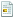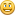September 21, 2023, 08:37:36 PM

## News:

Own IWBasic 2.x ? -----> Get your free upgrade to 3.x now.........

Started by Guilect, August 29, 2008, 07:26:53 PM

0 Members and 1 Guest are viewing this topic.

#### Guilect

#####August 29, 2008, 07:26:53 PM
I am aware of the 3DSprite commands, however I an trying to learn about Meshes.
I would like to create a '2D' mesh (aka billboard or sprite) then texurize it.
I have the following but it does not work,
it creates the mesh but the texture does not show.
help.......
`'//Example of creating a custom mesh.'//Handles ALT-TAB switching by using a restore callbackCONST DIK_UP = 0xC8 /* UpArrow on arrow keypad */CONST DIK_PRIOR = 0xC9 /* PgUp on arrow keypad */CONST DIK_LEFT = 0xCB /* LeftArrow on arrow keypad */CONST DIK_RIGHT = 0xCD /* RightArrow on arrow keypad */CONST DIK_END = 0xCF /* End on arrow keypad */CONST DIK_DOWN = 0xD0 /* DownArrow on arrow keypad */CONST DIK_NEXT = 0xD1 /* PgDn on arrow keypad */CONST DIK_Z =              0x2CCONST DIK_X =              0x2DCONST DIK_R =              0x13CONST DIK_ESCAPE =         0x01 C3DScreen s C3DCamera c C3dMesh m C3DObject scene C3DLight light float fadjust,fTarget '//target 60FPS for camera movement fTarget = 1.0f / /*FPS*/60.0f * 1000.0f fAdjust = 1.0f s.CreateWindowed(0,0,640,480,@CAPTION,"Custom Mesh",NULL,TRUE) c.Create(s) c.Position(0,0,-8) c.Orient(0,0,1,0,1,0) c.SetBackPlane(500) m.createmesh(s,4,6,D3DFVF_XYZ | D3DFVF_NORMAL | D3DFVF_DIFFUSE ) '//lock the buffers and write the vertices POINTER pVB POINTER pIB pVB = m.LockVertexBuffer() pIB = m.LockIndexBuffer() #<VERTEX0TEXTURE>pVB.position.x = -1 #<VERTEX0TEXTURE>pVB.position.y = -1 #<VERTEX0TEXTURE>pVB.position.z = 0 #<VERTEX0TEXTURE>pVB.diffuseColor = RGBA(255,255,255,255) #<VERTEX0TEXTURE>pVB.normal = Vec3Normalize(#<VERTEX0TEXTURE>pVB.position) pVB += len(VERTEX0TEXTURE) #<VERTEX0TEXTURE>pVB.position.x = 1 #<VERTEX0TEXTURE>pVB.position.y = -1 #<VERTEX0TEXTURE>pVB.position.z = 0 #<VERTEX0TEXTURE>pVB.diffuseColor = RGBA(255,255,255,255) #<VERTEX0TEXTURE>pVB.normal = Vec3Normalize(#<VERTEX0TEXTURE>pVB.position) pVB += len(VERTEX0TEXTURE) #<VERTEX0TEXTURE>pVB.position.x = -1 #<VERTEX0TEXTURE>pVB.position.y = 1 #<VERTEX0TEXTURE>pVB.position.z = 0 #<VERTEX0TEXTURE>pVB.diffuseColor = RGBA(255,255,255,255) #<VERTEX0TEXTURE>pVB.normal = Vec3Normalize(#<VERTEX0TEXTURE>pVB.position) pVB += len(VERTEX0TEXTURE) #<VERTEX0TEXTURE>pVB.position.x = 1 #<VERTEX0TEXTURE>pVB.position.y = 1 #<VERTEX0TEXTURE>pVB.position.z = 0 #<VERTEX0TEXTURE>pVB.diffuseColor = RGBA(255,255,255,255) #<VERTEX0TEXTURE>pVB.normal = Vec3Normalize(#<VERTEX0TEXTURE>pVB.position) pVB += len(VERTEX0TEXTURE)'//write the indices.  Each index is the zero based entry of the array of vertexes.  '  '  --------------'  \'  |\ '  |  \'  |   \'  |    \'  |     \'  |      \' --------------  ' '// indices #<WORD>pIB = 0 #<WORD>pIB = 2 #<WORD>pIB = 1 '// triangle #<WORD>pIB = 1 #<WORD>pIB = 2 #<WORD>pIB = 3 '//unlock the buffers m.UnlockIndexBuffer() m.UnlockVertexBuffer() m.setvisible(true) ' load in some bitmap m.loadtexture(0,"bug.bmp",0) light.Create(s,LIGHT_POINT,1) light.Position(0,20,-100) light.SetAttenuation(0,1/200.0,0) light.SetSpecular(.5,.5,.5,1) light.SetAmbient(.4,.4,.4,1) scene.CreateScene(s) scene.AddChild(light) scene.AddChild(m) int fps:fps = 0 int startTime do startTime = MILLISECS() '//check for keys and move camera accordingly IF KeyDown(DIK_UP) then c.Move(0.0f,.25f * fAdjust) IF KeyDown(DIK_DOWN) then c.Move(0.0f,-.25f * fAdjust) IF KeyDown(DIK_RIGHT) then c.Rotate(1*.01745 * fAdjust,0,0) IF KeyDown(DIK_LEFT) then c.Rotate(-1*.01745 * fAdjust,0,0) IF KeyDown(DIK_Z) then c.Rotate(0,0,-1 *.01745 * fAdjust) IF KeyDown(DIK_X) then c.Rotate(0,0,1 *.01745 * fAdjust) IF KeyDown(DIK_PRIOR) then c.Rotate(0,-1 *.01745 * fAdjust,0) IF KeyDown(DIK_NEXT) then c.Rotate(0,1 *.01745 * fAdjust,0) IF KeyDown(DIK_R) then c.LookAt(0,0,0) s.Clear(RGBA(0,0,0,255)) s.BeginScene(c) scene.Draw() s.RenderText(0,10,"ESC to exit",RGBA(255,255,0,200)) s.RenderText(0,30,"FPS:"+str\$(fps),RGBA(255,255,0,255)) fps = s.RenderScene() fAdjust = (MILLISECS() - startTime) / fTarget until KeyDown(DIK_ESCAPE) Scene.Free()END`

#### Barney

#####August 30, 2008, 04:41:28 AM #1
In order to have the object properly textured you need to setup the texture coordinates. While the texture coordinates for the built-in meshes like cube are already provided, texture coordinates for your own meshes are not created automatically. You have to create them yourself.

First you need to change the mesh creation command. It should look like this:

` m.createmesh(s,4,6,D3DFVF_XYZ | D3DFVF_NORMAL | D3DFVF_DIFFUSE | D3DFVF_TEX1)`

Note that I have added D3DFVF_TEX1 constant, which will make sure there's enough space reserved in the memory, not only for the basic mesh data but for texture coordinates as well.

Then you need to change all occurences of VERTEX0TEXTURE to VERTEX1TEXTURE and add the necessary lines with the tex coordinates:

` #<VERTEX1TEXTURE>pVB.position.x = -1 #<VERTEX1TEXTURE>pVB.position.y = -1 #<VERTEX1TEXTURE>pVB.position.z = 0 #<VERTEX1TEXTURE>pVB.diffuseColor = RGBA(255,255,255,255) #<VERTEX1TEXTURE>pVB.normal = Vec3Normalize(#<VERTEX1TEXTURE>pVB.position) #<VERTEX1TEXTURE>pVB.texCoords.x = 0 #<VERTEX1TEXTURE>pVB.texCoords.y = 0 pVB += len(VERTEX1TEXTURE) #<VERTEX1TEXTURE>pVB.position.x = 1 #<VERTEX1TEXTURE>pVB.position.y = -1 #<VERTEX1TEXTURE>pVB.position.z = 0 #<VERTEX1TEXTURE>pVB.diffuseColor = RGBA(255,255,255,255) #<VERTEX1TEXTURE>pVB.normal = Vec3Normalize(#<VERTEX1TEXTURE>pVB.position) #<VERTEX1TEXTURE>pVB.texCoords.x = 1 #<VERTEX1TEXTURE>pVB.texCoords.y = 0 pVB += len(VERTEX1TEXTURE) #<VERTEX1TEXTURE>pVB.position.x = -1 #<VERTEX1TEXTURE>pVB.position.y = 1 #<VERTEX1TEXTURE>pVB.position.z = 0 #<VERTEX1TEXTURE>pVB.diffuseColor = RGBA(255,255,255,255) #<VERTEX1TEXTURE>pVB.normal = Vec3Normalize(#<VERTEX1TEXTURE>pVB.position) #<VERTEX1TEXTURE>pVB.texCoords.x = 0 #<VERTEX1TEXTURE>pVB.texCoords.y = 1 pVB += len(VERTEX1TEXTURE) #<VERTEX1TEXTURE>pVB.position.x = 1 #<VERTEX1TEXTURE>pVB.position.y = 1 #<VERTEX1TEXTURE>pVB.position.z = 0 #<VERTEX1TEXTURE>pVB.diffuseColor = RGBA(255,255,255,255) #<VERTEX1TEXTURE>pVB.normal = Vec3Normalize(#<VERTEX1TEXTURE>pVB.position) #<VERTEX1TEXTURE>pVB.texCoords.x = 1 #<VERTEX1TEXTURE>pVB.texCoords.y = 1 pVB += len(VERTEX1TEXTURE)`

That's all there is to it.

Barney

#### Guilect

#####August 30, 2008, 07:27:19 AM #2
Thanks Barney.

QuoteThat's all there is to it.
Easy for you to say.